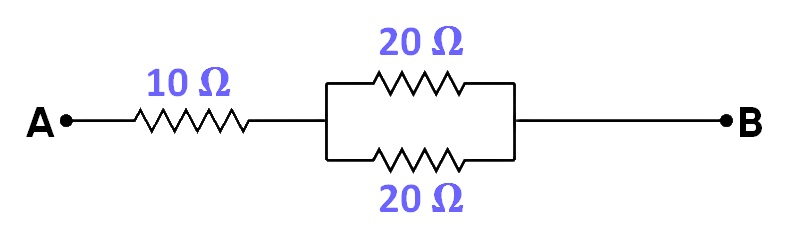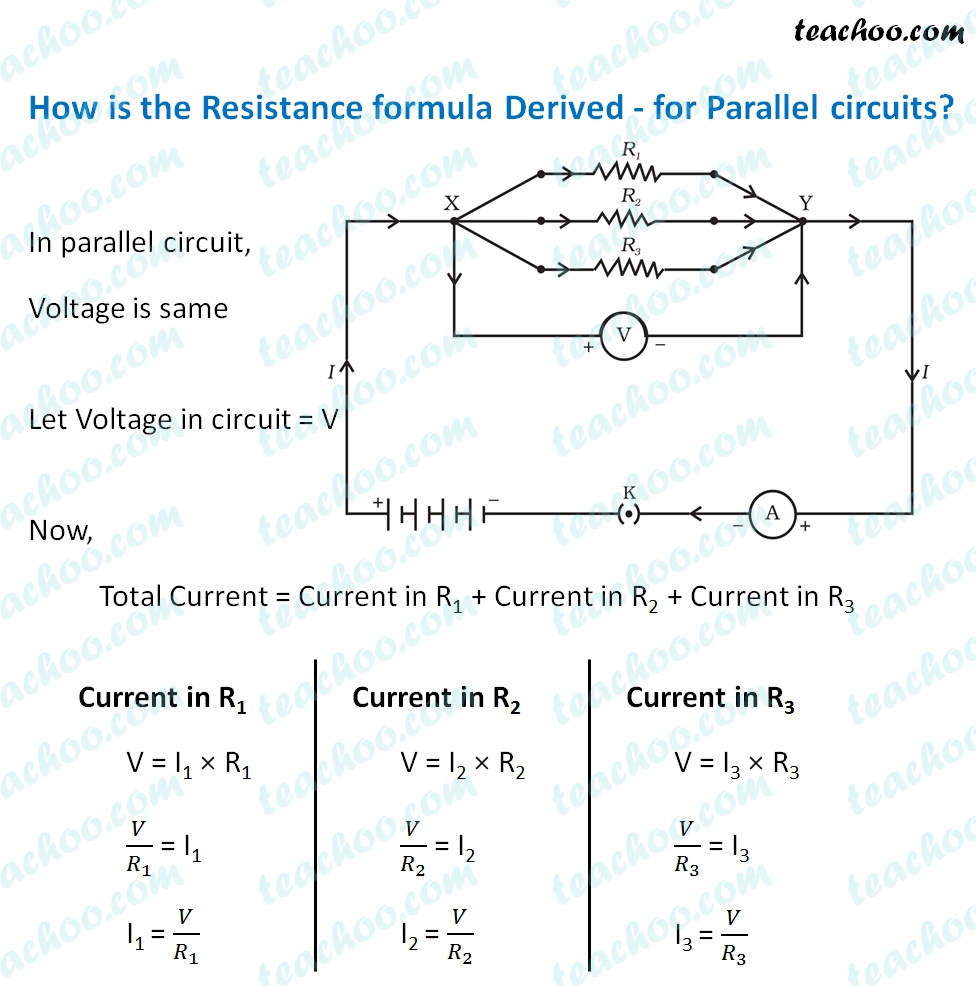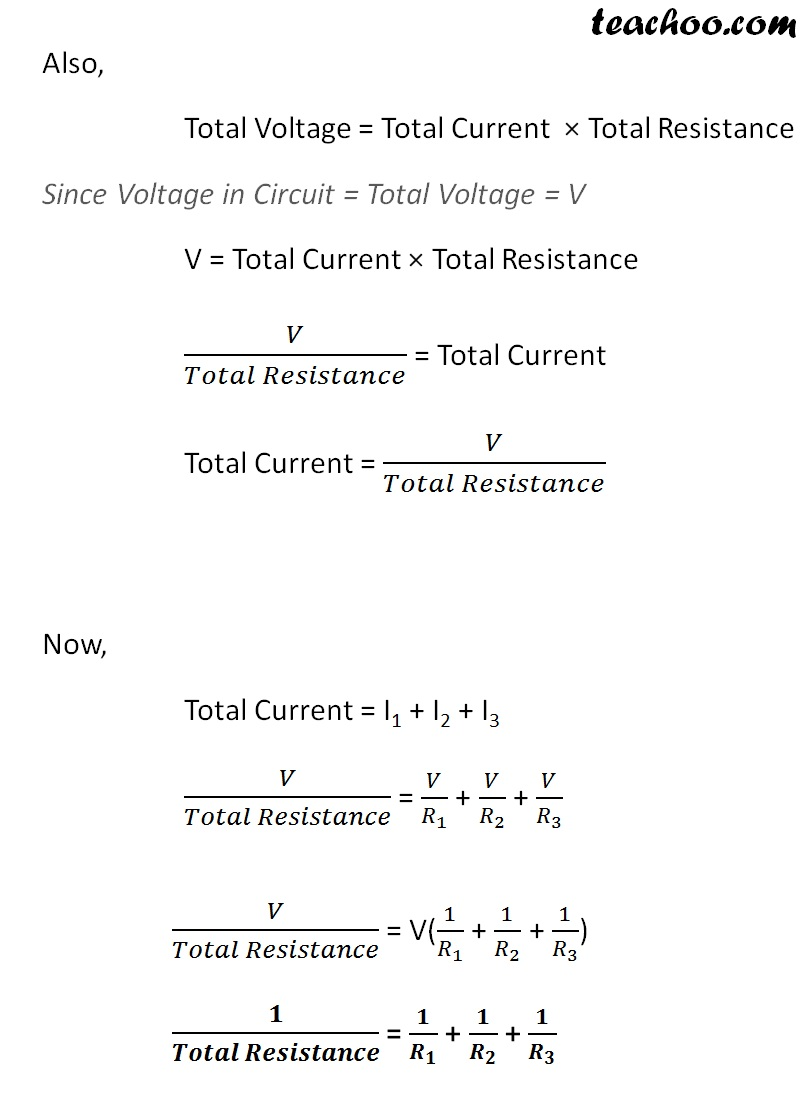Past Year - 5 Mark Questions

Class 10
Chapter 12 Class 10 - Electricity

## (b) Calculate the equivalent resistance of the following network:(a)Note - In case of parallel, the total resistance is always less than the resistance of the individual resistors.

The net resistance produced is lowest when resistors are connected in parallel.

(b)

Let R’ be the equivalent resistance of the two 20 ohm resistors connected in parallel.

Therefore,

1 / R’ = 1 / 20 + 1 / 20

= 2 / 20

R’ = 20 / 2 = 10 ohm

Let R be the equivalent resistance of the entire network.

The 10 ohm resistor and R’ are in series.

Therefore,

R = R’ + 10 ohm = 10 + 10 = 20 ohm

Therefore, the equivalent resistance of the given network is 20 ohm.

Learn in your speed, with individual attention - Teachoo Maths 1-on-1 Class